Courses

# Chemistry Test - 3

## 45 Questions MCQ Test | Chemistry Test - 3

Description
This mock test of Chemistry Test - 3 for helps you for every entrance exam. This contains 45 Multiple Choice Questions for Chemistry Test - 3 (mcq) to study with solutions a complete question bank. The solved questions answers in this Chemistry Test - 3 quiz give you a good mix of easy questions and tough questions. students definitely take this Chemistry Test - 3 exercise for a better result in the exam. You can find other Chemistry Test - 3 extra questions, long questions & short questions for on EduRev as well by searching above.
QUESTION: 1

Solution:
QUESTION: 2

Solution:
QUESTION: 3

### In the sulphonation of benzene, the active species involved is

Solution:
QUESTION: 4

The geometry of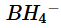unit is

Solution:
QUESTION: 5

The correct order of decreasing ionic character of lead dihalides is

Solution:
QUESTION: 6

Stable compounds in +1 oxidation state is formed by

Solution:
QUESTION: 7

The general trend in the properties of elements of carbon family shows that with the rise in atomic number

Solution:
QUESTION: 8

Which of the following statements is not correct ?

Solution:
QUESTION: 9

Which statement regarding H3BO3 is not correct ?

Solution:
QUESTION: 10

The different layers in graphite are held together by

Solution:
QUESTION: 11

Carbon monoxide acts as a Lewis base because it has

Solution:
QUESTION: 12

Number of structural isomers represented by molecular formula C4H10O is

Solution:
QUESTION: 13

The compound C4H10O can show

Solution:
QUESTION: 14

Which of the following are chain isomers ?

Solution:
QUESTION: 15

Number of isomers observed in C7H7Cl are

Solution:
QUESTION: 16

Which of the following compounds can exhibit both geometrical isomerism and enantiomerism ?

Solution:
QUESTION: 17

Which cannot be prepared by B2H6 ?

Solution:
QUESTION: 18

Aqueous solution of potash alum is

Solution:
QUESTION: 19

Which is a pair of geometrical isomers ?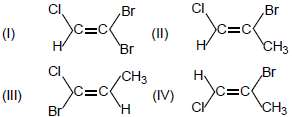Solution:
QUESTION: 20

Acetylene contains same degrees of unsaturation as

Solution:
QUESTION: 21

Which one of the following is the strongest acid ?

Solution:
QUESTION: 22

The number of electrons present in the valency shell of carbon of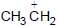ion bearing +ve charge

Solution:
QUESTION: 23

Which of the following is a pseudo alum ?

Solution:
QUESTION: 24

Stability of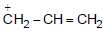can be explained by

Solution:
QUESTION: 25

Structure formula for lewisite is

Solution:
QUESTION: 26

Which of the following is the correct order of stability of free radicals ?

Solution:
QUESTION: 27

Arrange the following nucleophiles in the order of their nucleophilic strength

Solution:
QUESTION: 28

The increasing order of stability of the following free radicals is

Solution:
QUESTION: 29

Which of the following equations is not correctly formulated ?

Solution:
QUESTION: 30

Which of the following alkynes is most acidic ?

Solution:
QUESTION: 31

The compound obtained by the reaction of methyl alcohol with CH3MgX is

Solution:
QUESTION: 32

The number of different substitution products possible when bromine and ethane are allowed to react, is

Solution:
QUESTION: 33

Which of the following cannot be considered as a mechanistic step in chain reaction of methane with Cl2 ?

Solution:
QUESTION: 34

In the given reaction,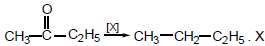Will be

Solution:
QUESTION: 35

What is formed when calcium carbide reacts with heavy water ?

Solution:
QUESTION: 36

Which of the following is the condition for best yield of C2H5Cl ?

Solution:
QUESTION: 37

Propene on ozonolysis forms

Solution:
QUESTION: 38

Which of the following statements regarding boron is not correct ?

Solution:
QUESTION: 39

The end product of the following sequence of operations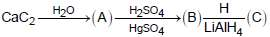Solution:
QUESTION: 40

The presence of unsaturation in an organic compound can be tested with

Solution:
QUESTION: 41

Relative stabilities of the following carbocations will be in the order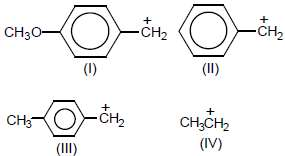Solution:
QUESTION: 42

CH2 = CH2 + HOCl → ....... + ...... → ethylene chlorohydrin. Fill in the blanks in the above reaction with

Solution:
QUESTION: 43

In the nitration of benzene with a mixture of conc.HNO3 and conc.H2SO4 , the active species involved is

Solution:
QUESTION: 44

The stability of +2 oxidation state of Pb can be explained on the basis of

Solution:
QUESTION: 45

The reaction of chlorine with toleune in presence of ferric chloride gives predominantly

Solution: